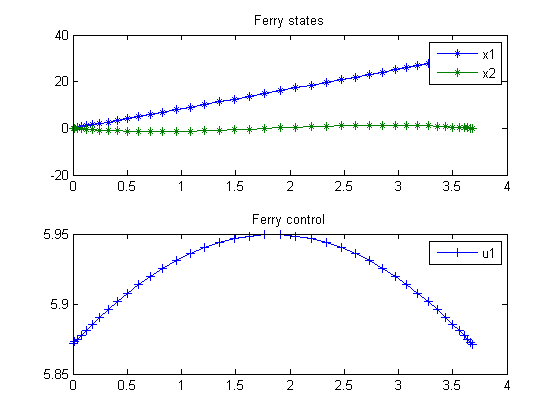126  Zermelos problem (version 2)

126.1  Problem description

Time-optimal crossing by boat of a river with a position dependent current stream.

Applied Optimal Control, Bryson & Ho, 1975. Example 1 on page 77.

Programmers: Gerard Van Willigenburg (Wageningen University) Willem De Koning (retired from Delft University of Technology)

126.2  Problem setup

% Array with consecutive number of collocation points
narr = [20 40];

toms t t_f % Free final time

for n=narr
p = tomPhase('p', t, 0, t_f, n);
setPhase(p)

tomStates x1 x2
tomControls u1

% Initial & terminal states
xi = [0;  0];
xf = [31; 0];

% Initial guess
if n==narr(1)
x0 = {t_f == 2; icollocate({x1 == xi(1); x2 == xi(2)})
collocate({u1 == 0})};
else
x0 = {t_f == tfopt; icollocate({x1 == xopt1; x2 == xopt2})
collocate({u1 == uopt1})};
end

% Box constraints
cbox = {1 <= t_f <= 10};

% Boundary constraints
cbnd = {initial({x1 == xi(1); x2 == xi(2)});
final({x1 == xf(1); x2 == xf(2)})};

% ODEs and path constraints
v = 9;
% No water motion in x1 direction
dx1 = v*cos(u1);
% Water motion in x2 direction: 5*sin(pi*x1/31)
dx2 = v*sin(u1)+5*sin(pi*x1/31);

ceq = collocate({
dot(x1) == dx1
dot(x2) == dx2});

% Objective
objective = t_f;

126.3  Solve the problem

options = struct;
options.name = 'Ferry trajectory optimization';
solution = ezsolve(objective, {cbox, cbnd, ceq}, x0, options);

tfopt = subs(t_f,solution);
xopt1 = subs(x1,solution);
xopt2 = subs(x2,solution);
uopt1 = subs(u1,solution);
Problem type appears to be: lpcon
Starting numeric solver
===== * * * =================================================================== * * *
TOMLAB - Tomlab Optimization Inc. Development license  999001. Valid to 2011-02-05
=====================================================================================
Problem: ---  1: Ferry trajectory optimization  f_k       3.681324334091522500
sum(|constr|)      0.000000334501118908
f(x_k) + sum(|constr|)      3.681324668592641300
f(x_0)      2.000000000000000000

Solver: snopt.  EXIT=0.  INFORM=1.
SNOPT 7.2-5 NLP code
Optimality conditions satisfied

FuncEv    1 ConstrEv   84 ConJacEv   84 Iter   51 MinorIter   99
CPU time: 0.156250 sec. Elapsed time: 0.156000 sec.
Problem type appears to be: lpcon
Starting numeric solver
===== * * * =================================================================== * * *
TOMLAB - Tomlab Optimization Inc. Development license  999001. Valid to 2011-02-05
=====================================================================================
Problem: ---  1: Ferry trajectory optimization  f_k       3.681324335373935800
sum(|constr|)      0.000002472599377322
f(x_k) + sum(|constr|)      3.681326807973313000
f(x_0)      3.681324334091522500

Solver: snopt.  EXIT=0.  INFORM=1.
SNOPT 7.2-5 NLP code
Optimality conditions satisfied

FuncEv    1 ConstrEv   40 ConJacEv   40 Iter   32 MinorIter  112
CPU time: 0.203125 sec. Elapsed time: 0.203000 sec.
end

% Get solution
t  = subs(collocate(t),solution);
x1 = subs(collocate(x1),solution);
x2 = subs(collocate(x2),solution);
u1 = subs(collocate(u1),solution);

%Bound u1 to [0,2pi]
u1 = rem(u1,2*pi); u1 = (u1<0)*2*pi+u1;

% Plot final solution
figure(1)
subplot(2,1,1)
plot(t,x1,'*-',t,x2,'*-');
legend('x1','x2');
title('Ferry states');

subplot(2,1,2)
plot(t,u1,'+-');
legend('u1');
title('Ferry control');